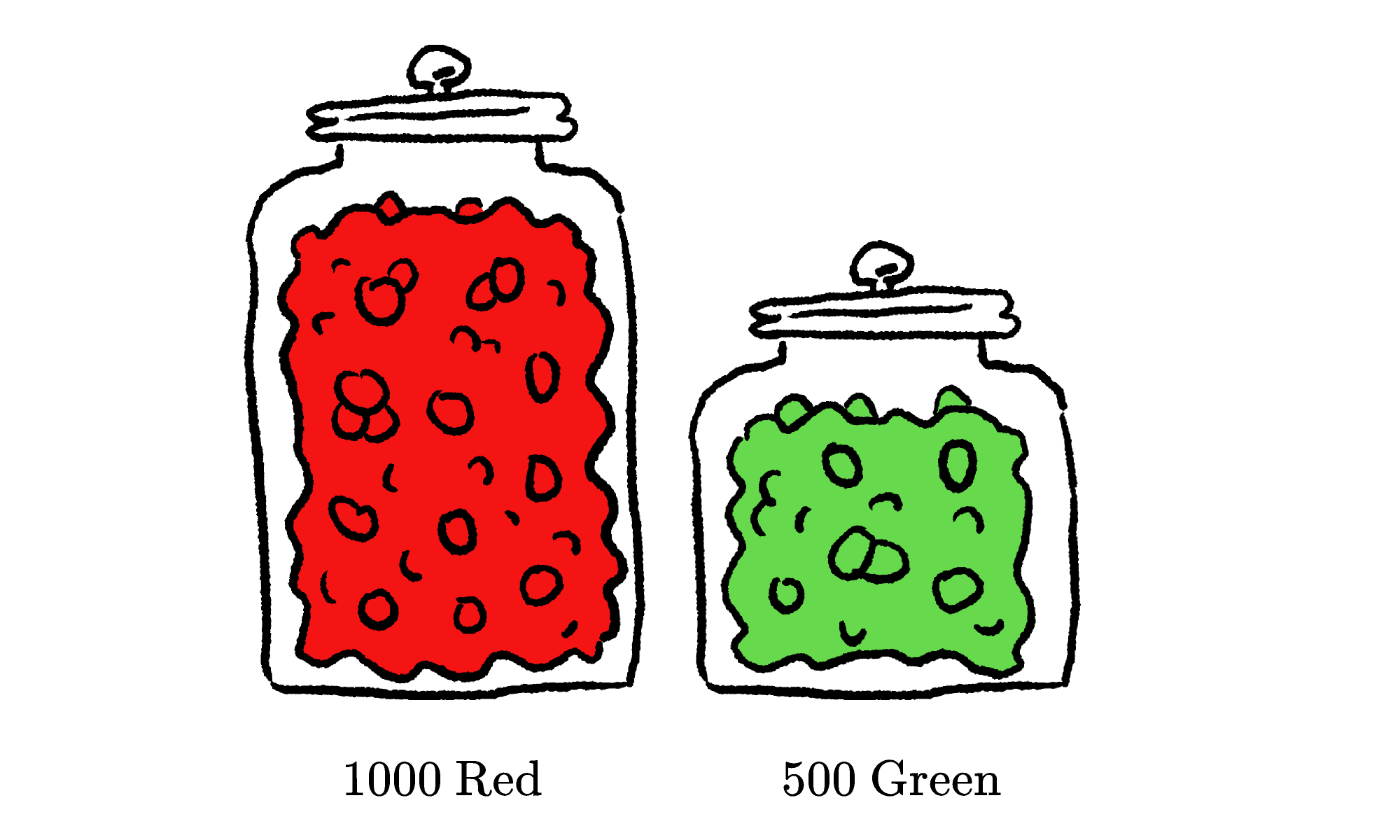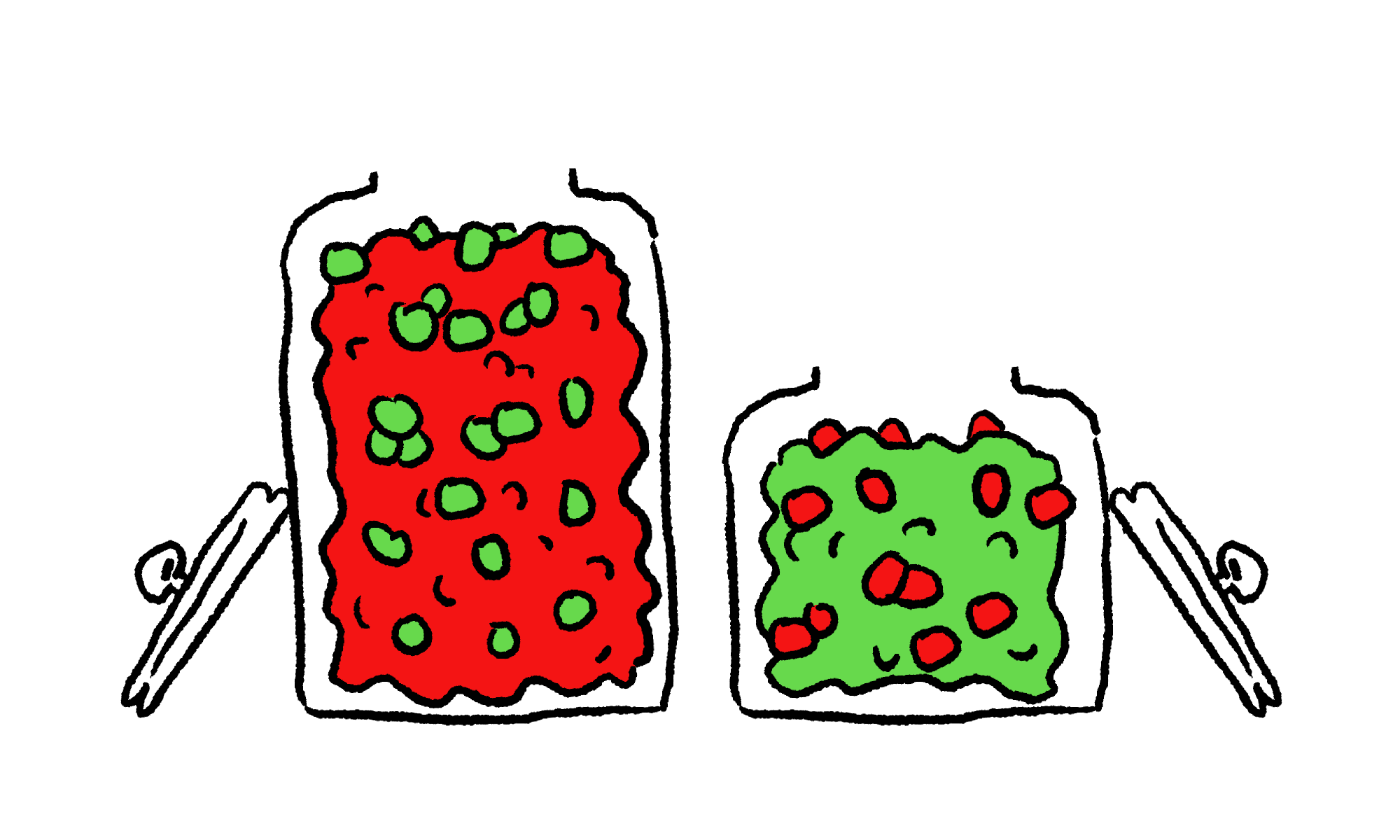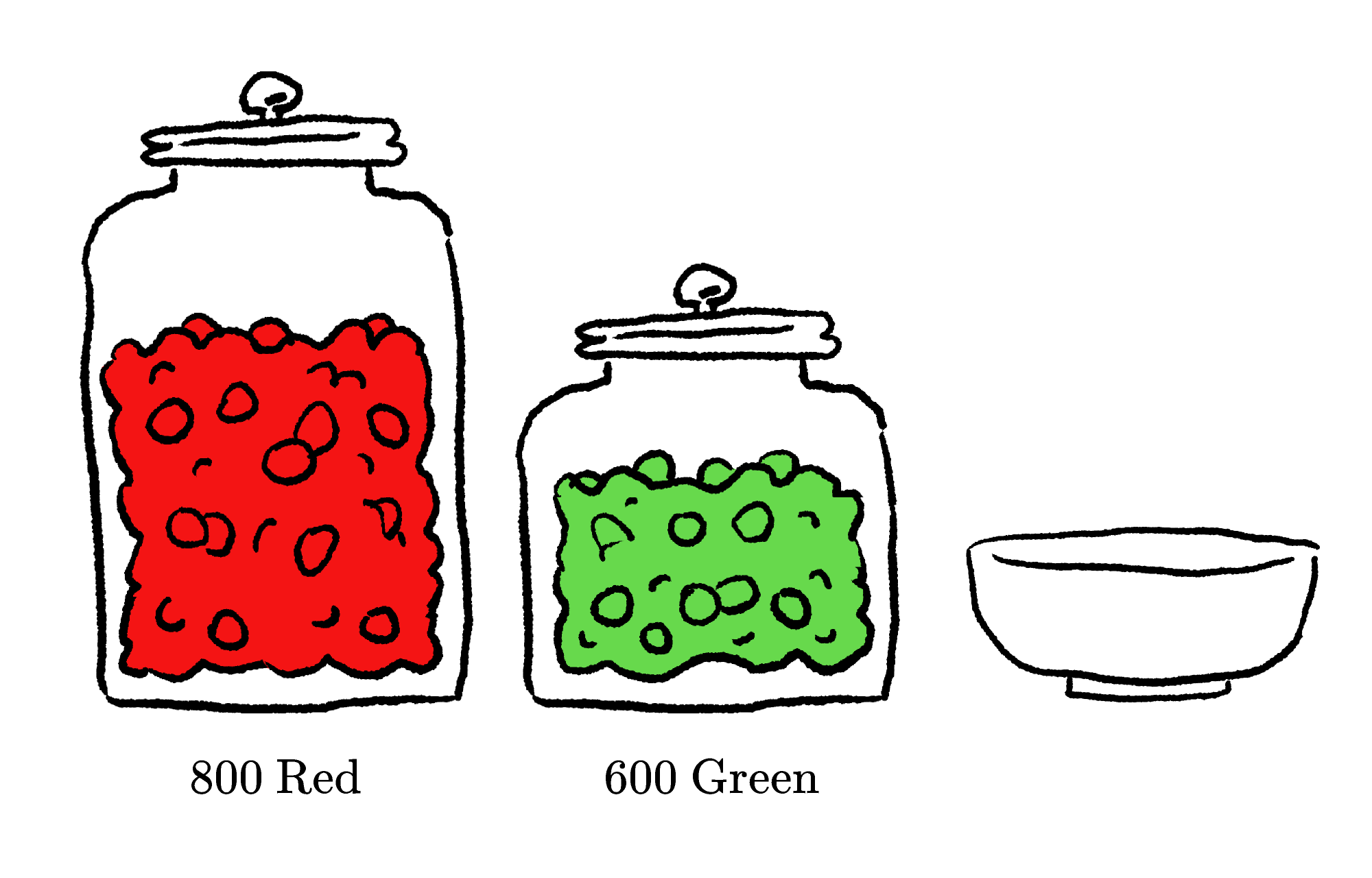Back

## The Great Candy Mixup

Below are two jars of candies, with $1000$ red candies in one and $500$ green candies in the other.Let's say we take $200$ red candies from the large jar, thoroughly mix them into the small jar, and then take $200$ random candies from the small jar and put them in the large jar. Which jar now has a higher concentration of the candies it started with? Keep reading to find out, or jump ahead to today's challenge.

After all of the mixing, the two jars will look something like this:Let's say we ended up with $x$ red candies in the small jar. We know each jar ends up with the same number of candies it started with, so those $x$ candies must have replaced $x$ green candies. This means there must be exactly $x$ green candies in the right jar, and both jars have been equally "contaminated" by the other color if we're only considering the number of "wrong" candies.

What if we think about this contamination relative to the sizes of the jars, though? The small jar has $500$ candies, so $\frac{x}{500}$ of its candies are now red instead of green. Meanwhile, $\frac{x}{1000}$ of the large jar's candies are green instead of red. Looking at things this way, the small jar has been affected twice as intensely as the larger jar.

For example, let's say the large jar ends up with $182$ green candies and $818$ red candies. This means the small jar must have $182$ red candies and $318$ green candies, since equal numbers of candies have been swapped. Although the number of wrong-colored candies is the same, the percentage contamination to the larger jar is $\frac{182}{1000} = 18.2\%$ and the percentage contamination to the smaller jar is $\frac{182}{500} = 36.4\%.$ The smaller jar's contamination is twice as large relative to the size. This effect would have been magnified even more had the small jar been smaller!

# Today's Challenge

Jar A has 800 red candies and jar B has 600 green candies.If we do the following...

1. Move one cup of red candies from jar A to jar B and mix.
2. Move one cup of mixed candies from jar B to jar A and mix.
3. Move one cup of candies from each of jar A and jar B to the bowl and mix.
4. Randomly select one candy from the bowl.

...is the candy we select more likely to be red or green?×